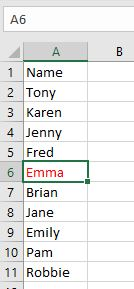# VBA Find Range in Excel (Range.Find)

Range.Find in VBA is the same as using the Find command on the Excel Ribbon, but within our VBA Code.

## Range.Find Syntax

The VBA Find function uses the Range object to search for a specified value in a Range of cells provided.  When you use the VBA Range.Find Function, you provide the function with parameters, the syntax of which is shown below: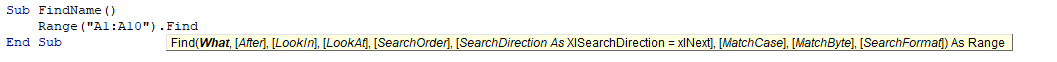The only required parameter is What your are looking for.  The rest of the parameters enable you to narrow down you search based on the criteria set in the parameters.

 Parameter Type Description Values What Required The value you are searching for Any data After Optional A single cell range that you start your search from for example: Range(“A5”) LookIn Optional What to search in eg: Formulas, Values or Comments xlValues, xlFormulas, xlCommentsThreaded, xlNotes LookAt Optional Look at a part or the whole of the cell xlWhole, xlPart SearchOrder Optional The order to search xlByRows or xlByColumns. SearchDirection Optional The direction to search xlNext, xlPrevious MatchCase Optional If search is case sensitive True or False MatchByte Optional Used for double byte languages True or False SearchFormat Optional Allow searching by format. The format is set using Application.FindFormat True or False

## Range.Find What

As mentioned above, all the parameters except the What parameter are optional. Therefore, you can find the word “Tony” in the Range (“A1:A10”) by running the simple macro below.  We can then select the found value by going to the cell where the word is found (rng.Select).

``````Sub FindName()
Dim rng As Range
Set rng = Range("A1:A10").Find("Tony")
If Not rng Is Nothing Then rng.Select
End Sub``````

## Range.Find After

If we wish to start searching for the word at a specific location, we can use the After parameter.  The macro below will therefore only start searching for the word ‘Tony’ after cell A3 in the Range (“A1:A10”).

``````Sub FindNameAfter()
Dim rng As Range
Set rng = Range("A1:A10").Find("Tony", Range("A3"))
If Not rng Is Nothing Then rng.Select
rng.Select
End Sub``````

## Range.Find LookIn

The Look In argument specifies to either look in the formula contained in the cell, in the value contained in the cell or in any comment/note that may be attached to a cell in the specified range that is being searched.

``````Sub FindComment()
Dim rng As Range
If Not rng Is Nothing Then rng.Select
End Sub``````

Note that we have left an empty parameter between the What and the LookIn parameters.  If you do not want to leave this empty parameter, then you need to use named parameters in your code.  The first parameter does not need naming however.

We can name the parameter as shown below:

``````Sub FindComment()
Dim rng As Range
If Not rng Is Nothing Then rng.Select
End Sub``````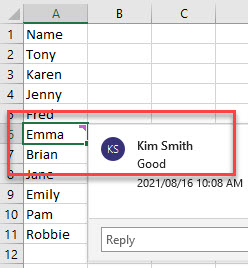## Range.Find LookAt

The Look At parameter gives you the option to find all cells that contain a certain part of the value you are looking for.

``````Sub FindComment()
Dim rng As Range
Set rng = Range("A1:A10").Find("Em", LookIn:=xlValues, Lookat:=xlPart)
If Not rng Is Nothing Then rng.Select
End Sub``````

The code example below will first find the name “Emma” as it starts with “Em”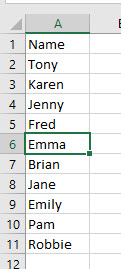If we were to amend the code slightly to start looking from A7, then the name “Emily” would be found instead.

``````Sub FindComment()
Dim rng As Range
Set rng = Range("A1:A10").Find("Em", After:=Range("A3"), LookIn:=xlValues, Lookat:=xlPart)
If Not rng Is Nothing Then rng.Select
End Sub``````

## Range.Find SearchOrder

The Search Order parameter  tells Excel to look first by Rows and then by Columns, or the other way around.  The example below will look down column A, and then start looking in Column B to find the value required.

``````Sub FindComment()
Dim rng As Range
Set rng = Range("A1:B10").Find("Emily", SearchOrder:=xlColumns)
If Not rng Is Nothing Then rng.Select
End Sub``````

This example however, will look in A1 and B1, and then in A2 and B2, and so on – in other words, it will search by Row.

``````Sub FindComment()
Dim rng As Range
Set rng = Range("A1:A10").Find("Emily", SearchOrder:=xlRows)
If Not rng Is Nothing Then rng.Select
End Sub``````

Stop searching for VBA code online. Learn more about AutoMacro - A VBA Code Builder that allows beginners to code procedures from scratch with minimal coding knowledge and with many time-saving features for all users!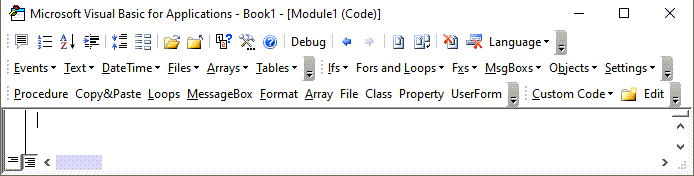## Range.Find SearchDirection

The Search Direction parameter enables us to search forwards or backwards.   For example, the code below would find Emily before it finds Emma as it starts the search at cell A10 and search backwards until it finds a match.  Using xlNext will start the search at A1 and sort forwards until it finds a match. It it finds a match, it will select the cell where the match is.

``````Sub FindComment()
Dim rng As Range
Set rng = Range("A1:A10").Find("Em", SearchDirection:=xlPrevious)
If Not rng Is Nothing Then rng.Select
End Sub``````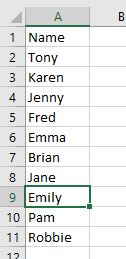## Range.Find MatchCase

The Match Case parameter enables us to search for an exact match based on upper, lower or proper case.

``````Sub MatchCase()
Dim rng As Range
Set rng = Range("A1:A10").Find("em",  Lookat:=xlPart, MatchCase:=True)
If Not rng Is Nothing Then rng.Select
End Sub``````

The code above will not find anything as there is “Emma” and “Emily” in the data, but both begin with a capital “E”.

``````Sub MatchCase()
Dim rng As Range
Set rng = Range("A1:A10").Find("Em", Lookat:=xlPart, MatchCase:=True)
If Not rng Is Nothing Then rng.Select
End Sub``````

## Range.Find MatchByte

This parameter is only used with languages with a double-byte character set such as Japanese or Korean – it is not relevant to this article.

VBA Programming | Code Generator does work for you!

## Range.Find SearchFormat

The Search Format parameter enables us to search for a specific format of a cell – such as background color or a bold format.

We first need to set the FindFormat Property of the Application object, and then set the SearchFormat parameter to True.

``````Sub FindFormat()
Application.FindFormat.Clear
Application.FindFormat.Font.Color = vbRed
Dim rng As Range
Set rng = Range("A1:A10").Find("em", SearchFormat:=True)
If Not rng Is Nothing Then rng.Select
End Sub``````